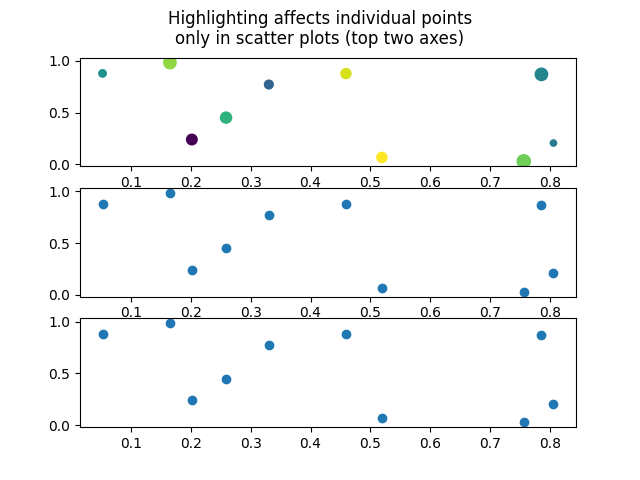# Scatter plots are highlighted point-by-point.¶

... as opposed to lines with a `"."` style, which have the same appearance, but are highlighted as a whole.```import numpy as np
import matplotlib.pyplot as plt
import mplcursors

x, y, z = np.random.random((3, 10))
fig, axs = plt.subplots(3)
fig.suptitle("Highlighting affects individual points\n"
"only in scatter plots (top two axes)")
axs.scatter(x, y, c=z, s=100 * np.random.random(10))
axs.scatter(x, y)
axs.plot(x, y, "o")
mplcursors.cursor(highlight=True)
plt.show()
```

Gallery generated by Sphinx-Gallery